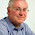## Wednesday, 6 May 2009

### Breaking Loan Payments Into Principal and Interest Components

By Stephen Nelson

Microsoft Excel can help you down a loan payment into its principal and interest components. Excel's IPMT function lets you calculate the interest component of a loan payment. And Excel's PPMT function lets you calculate the principal component of a payment.

Using the IPMT Function to Calculate Payment Interest

The IPMT function calculates the interest portion of a payment given its interest rate, the period, the term (or number of payments), present value (or loan balance), future value (or balloon payment), and, optionally, the type-of-annuity switch. If you set the type-ofannuity switch to 1, Excel assumes payments occur at the beginning of the period, following the annuity due convention. If you set the annuity switch to 0 or you omit the argument, Excel assumes payments occur at the end of the period following the ordinary annuity convention.

The function uses the following syntax:

IPMT (rate, period, nper, pv, fv, type)

For example, to calculate the period interest rate for the 54th payment on a 30-year, \$150,000 mortgage charging 8% annual interest, you use the following formula:

=IPMT(.08/12,54,30*12,150000,0,0)

The function returns the value -957.51. Notice that to convert the 8% annual interest to a period interest, the formula divides the annual interest rate by 12. Notice, too, that to convert the 30-year term to a term in months, the formula multiplies 30 by 12. The function returns the interest payment amount as a negative value because it reflects a cash outflow you pay.

NOTE If you set the pv argument to -150000, you indicate that you're loaning money. In this case, the function returns 957.51, a positive value, showing that the interest payment amount is a positive cash inflow.

Using the PPMT Function to Calculate Payment Principal

The PPMT function calculates the principal portion of a payment given its interest rate, the period, the term (or number of payments), present value (or loan balance), future value (or balloon payment), and, optionally, the type-of-annuity switch. If you set the type-of-annuity switch to 1, Excel assumes payments occur at the beginning of the period, following the annuity due convention. If you set the annuity switch to 0 or you omit the argument, Excel assumes payments occur at the end of the period following the ordinary annuity convention. The function uses the following syntax:

PPMT (rate, period, nper, pv, fv, type)

For example, to calculate the period principal payment for the 54th payment on a 30-year, \$150,000 mortgage charging 8% annual interest, you use the following formula:

=PPMT (.08/12,54,30*12,150000,0,0)

The function returns the value -143.13. Notice that to convert the 8% annual interest to a period interest, the formula divides the annual interest rate by 12. Notice, too, that to convert the 30-year term to a term in months, the formula multiplies 30 by 12. The function returns the principal payment amount as a negative value because it reflects a cash outflow you pay.

NOTE: If you set the pv argument to -150000, you indicate that you're actually loaning money. And in this case, the function returns 143.13, a positive value, showing that the principal payment amount is a positive cash inflow.

Stephen L. Nelson is a Bellevue, Washington CPA and the author of many bestselling books including the MBA's Guide to Microsoft Excel from which this article is adapted. Nelson also edits the popular information web sites forming an S corp online and the incorporating a business, the forming an LLC web sites.
Article Source:
http://EzineArticles.com/?expert=Stephen_Nelsonhttp://EzineArticles.com/?Breaking-Loan-Payments-Into-Principal-and-Interest-Components&id=859513

1.Thank you so much!!! I' am currently a real estate and finance graduate student. Your post and your instructions are so easy to follow. You have simplified so many formulas to calculate payments, interest, principal paid to date etc... Into straight to the point illustration and table! I don't know why they don't teach this in school first then the formulas and theory as supplemental lectures... Thanks once again, I was doing all these step by step on paper :)
>>>>>>>>>>~~~~~~~~~~~~>>>>>>>>>>>
secured loans

2.The IPMT function calculates the interest portion of a payment given its interest rate, the period, the term (or number of payments), Credit Restoration present value (or loan balance), future value (or balloon payment), and, optionally, the type-of-annuity switch.

3.And Excel's PPMT function lets you calculate the principal component of a payment. Ian Hepworth

4.The function returns the interest payment amount as a negative value because it reflects a cash outflow you pay. Dave

5.If you set the annuity switch to 0 or you omit the argument, Excel assumes payments occur at the end of the period following the ordinary annuity convention. canadian pay day loans

6.This comment has been removed by the author.

7.If you set the annuity switch to 0 or you omit the argument, Excel assumes payments occur at the end of the period following the ordinary annuity convention.låna

8.Excel's IPMT function lets you calculate the interest component of a loan payment. And Excel's PPMT function lets you calculate the principal component of a payment. freecreditreport